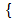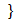# Upcoming Talks# Cohomological Hall algebras of Higgs torsion sheaves and moduli of stable triples

Date: Thursday, January 18, 2018 13:00 - 15:00
Speaker: Alexander Minets (Université Paris-Sud)
Location: Big Seminar room Ground floor / Office Bldg West (I21.EG.101)
Series: Mathematics and CS Seminar
Host: Tamas HauselGiven a (hereditary) finitary abelian category $\mathcal C$, one can associate to it the Hall algebra $H_\mathcal C$, which encodes the combinatorial information about extensions between objects in $\mathcal C$. When $\mathcal C$ is the category of finite-dimensional representations of a quiver over a finite field, these algebras are rather well understood and provide a useful viewpoint on quantum groups. Much less is known when $\mathcal C=\operatornameCohX$ is the category of coherent sheaves over a smooth projective curve $X$, even though they seem to encode interesting geometric and arithmetic data associated to curves, such as the number of cuspidal functions. In particular, there is no comprehensive representation theory of such algebras.

My talk will consist of two parts. The first hour will primarily serve as a motivation for the second part, and will be dedicated to an overview of the classical theory of Hall algebras. We will recall their relation to quantum groups as evidenced by Ringel's theorem, and some structural results in the case when $\mathcal C=\operatornameCohX$. In the second hour, I will shift the gears somewhat and introduce the cohomological version of Hall algebras (so-called CoHAs). I will then discuss some recent results providing an action of CoHA of Higgs torsion sheaves on homology groups of certain moduli spaces. These moduli spaces are heavily inspired by Nakajima quiver varieties, and are closely related to Hilbert schemes of points and moduli of sheaves on $T^*X$. If time permits, I will speculate about possible analogs of these CoHAs for an arbitrary smooth surface $S$ and/or extension of these results to Higgs bundles.